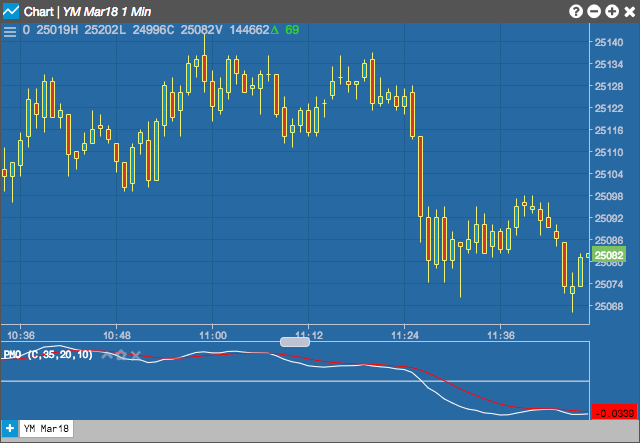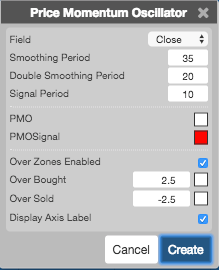Charts

# Price Momentum Oscillator

The Price Momentum Oscillator (PMO) indicator compares the current price with the previous price from a selected number of periods ago. This indicator is similar to the “Rate of Change” indicator, but the MOM does not normalize the price, so different instruments can have different indicator values based on their point values.## Configuration Options• Field: Price or combination of prices to use as the base for average calculations. Possible values include:
• Open
• High
• Low
• Close
• HL/2 $$\left ( \frac{High + Low}{2} \right )$$
• HLC/3 $$\left ( \frac{High + Low + Close}{3} \right )$$
• HLCC/4 $$\left ( \frac{High + Low + Close + Close}{4} \right )$$
• OHLC/4 $$\left ( \frac{Open + High + Low + Close}{4} \right )$$